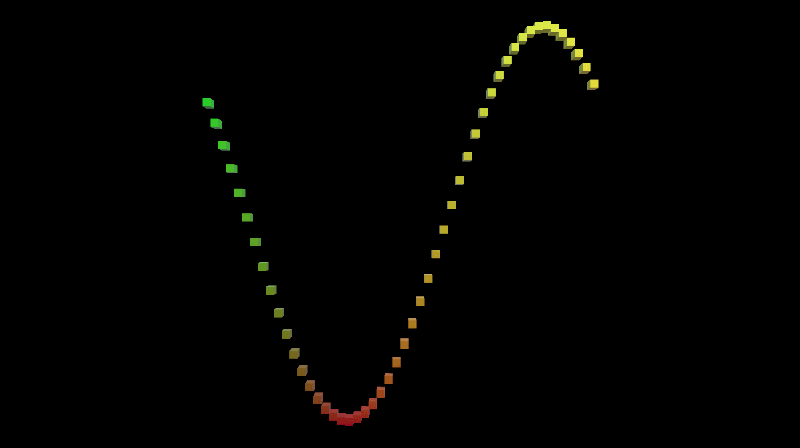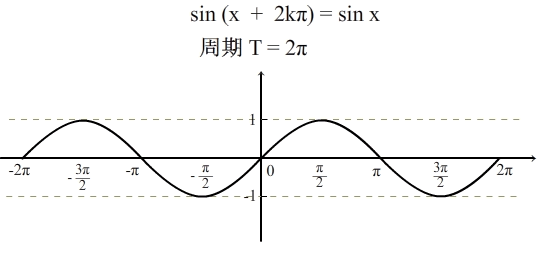Sin 函数是一个周期函数，也就是说，按照一定的长度去将这个图形切成一段一段的，那每一段都是相同的，而这个长度，就是2π。就像上图中的从 -π 到 π 就是一个周期。假设我们把这条 Sin 函数的曲线看成由一个个很小的点连起来构成的，而每一个点的位置，可以表示成 (x, y)，很明显，x 的范围就是 [-π , π]，而每一个点 y 的值是由 Sin(x) 算出来的。

using System.Collections;
using System.Collections.Generic;
using UnityEngine;

public class Graph : MonoBehaviour
{
// 建立一个 Cube
public Transform pointPrefab;

// 设定一个值，表示要用多少个 Cube 表示出这条曲线来，值越大，点就越细
[Range(10, 100)]
public int resolution = 10;

private Transform[] points;

private void Awake()
{
// 求出步长，相当于每一个 cube 的 x 轴相距多远
float step = 2f / resolution;

// 缩放一个 Cube
Vector3 scale = Vector3.one * step;

Vector3 position;
position.y = 0;
position.z = 0f;

points = new Transform[resolution];
for(int i = 0; i < resolution; ++i)
{
Transform point = Instantiate(pointPrefab);
// 将 x 轴限制在 [-1, 1] 之间，这里 + 0.5是偏移半个方块
position.x = (i + 0.5f) * step - 1f;
point.localPosition = position;
point.localScale = scale;
point.SetParent(transform, false);
points[i] = point;
}
}

private void Update()
{
for(int i = 0; i < points.Length; ++i)
{
Transform point = points[i];
Vector3 position = point.localPosition;

// 每个方块的 x 轴是确定的，y 轴的计算就是不断地用 x 轴坐标加上时间增量，
// 来做到周期移动的效果。这里之乘以 Mathf.PI 是为了将整个图像压缩在一定范围
position.y = Mathf.Sin((position.x + Time.time) * Mathf.PI);
point.localPosition = position;
}
}
}


o.Albedo.rg = IN.worldPos.xy * 0.5 + 0.5; 这一句就是根据坐标值设定颜色的 Shader 代码。这里为什么要乘 0.5 加 0.5 呢？因为上面的C#代码中，我们将每一个 Cube 的位置设置为了[-1, 1]之间，但是颜色的值不能为负，所以这里的乘 0.5 加 0.5 其实就是将坐标值映射为 [0, 1] 之间，从而作为颜色值来用。

Shader "Custom/ColoredPoint"
{
Properties
{
_Glossiness ("Smoothness", Range(0,1)) = 0.5
_Metallic ("Metallic", Range(0,1)) = 0.0
}
{
Tags { "RenderType"="Opaque" }
LOD 200

CGPROGRAM
// Physically based Standard lighting model, and enable shadows on all light types
#pragma surface surf Standard fullforwardshadows

// Use shader model 3.0 target, to get nicer looking lighting
#pragma target 3.0

struct Input
{
// 这一句是我们自己写的
float3 worldPos;
};

half _Glossiness;
half _Metallic;

// Add instancing support for this shader. You need to check 'Enable Instancing' on materials that use the shader.
// #pragma instancing_options assumeuniformscaling
UNITY_INSTANCING_BUFFER_START(Props)
// put more per-instance properties here
UNITY_INSTANCING_BUFFER_END(Props)

void surf (Input IN, inout SurfaceOutputStandard o)
{
// 这一句也是我们自己写的
o.Albedo.rg = IN.worldPos.xy * 0.5 + 0.5;

// Metallic and smoothness come from slider variables
o.Metallic = _Metallic;
o.Smoothness = _Glossiness;

// 这一句也是我们自己写的
o.Alpha = 1;
}
ENDCG
}
FallBack "Diffuse"
}